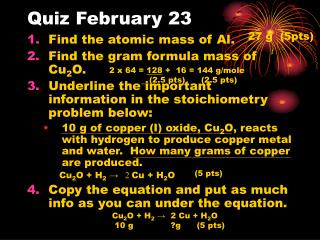DownloadDownload PresentationQuiz February 23

# Quiz February 23

Download Presentation## Quiz February 23

- - - - - - - - - - - - - - - - - - - - - - - - - - - E N D - - - - - - - - - - - - - - - - - - - - - - - - - - -
##### Presentation Transcript

1. Quiz February 23 27 g (5pts) 2 x 64 = 128 + 16 = 144 g/mole (2.5 pts) (2.5 pts) • Find the atomic mass of Al. • Find the gram formula mass of Cu2O. • Underline the important information in the stoichiometry problem below: • 10 g of copper (I) oxide, Cu2O, reacts with hydrogen to produce copper metal and water. How many grams of copper are produced. Cu2O + H2→ 2 Cu + H2O • Copy the equation and put as much info as you can under the equation. (5 pts) • Cu2O + H2 → 2 Cu + H2O • 10 g ?g (5 pts)

2. 15 g of copper (II) oxide,CuO, reacts with hydrogen to produce copper metal and water. How many grams of copper are produced.Cu2O + H2→ 2 Cu + H2O 1 2 15 g ?g = 13.3 g Cu 2Cu = 2x 64 = 128 O = 16 144g/mole 144g/mole x 64 g/mole 0.104 mole 0.208 mole

3. 29. Ammonia is synthesized from hydrogen and nitrogen according to the following equation: N2 + 3 H2→ 2NH3 If an excess of nitrogen is reacted with 3.41 g of hydrogen, how many grams of ammonia can be produced. N2 + 3 H2→ 2 NH3 3 2 N = 14 3H = 3 17 g/mole ?g = 19.3 g 3.41 g x 17g/mole 2 g/mole H2 1.7mole 1.14 mole

4. 30. Assume that in the decomposition of potassium chlorate, KClO3, 80.5 g of O2 form. How many grams of potassium chloride the other product forms. 2 2 K+Cl- + O2 2 KClO3 3 3 124 g = ?g 80.5 g 32 g/mole x 74 g/mole K =39 Cl = 35 74g/mole 2.52 mole 1.68 mole

5. 31. In a single displacement reaction, 9.23 g of aluminum react with excess hydrochloric acid. How many grams of hydrogen will be produced? 2 Al + 6 HCl  3 H2 + AlCl3 + Al3+Cl1- 2 2 3 9.23 g ? g = 1.03 g 2 H= 2x1 = 2  27g/mole 2 g/mole 0.34 mole 0.51 mole

6. Finish the Following for homework #32 p and 59,60 page 245 32. The compound “cisplatin” PtCl2(NH3)2 , has been found to be effective in treating some types of cancer. It can be synthesized using the reaction on page 237 of your book. a) How much “cisplatin” can be produced from 2.50 g PtCl2(NH3)2 and excess NH3. ? b) How much NH3 is needed? 59. In the decomposition of sodium chlorate, 31.7 g of oxygen are formed. How many grams of sodium chloride are produced? NaClO3 -> NaCl + O2 (unbalanced reaction) 60. The action of carbon monoxide on iron (III) oxide can be represented by Fe2O3 + 3 CO -> 2 Fe + 3 CO2. How much carbon monoxide is used if 57.5 g of iron were produced?